# Creating Math Equations

You can create editable math equation objects and place them in your documents.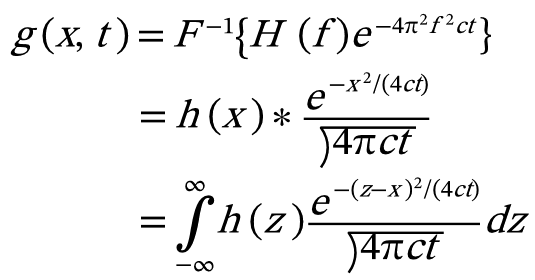#### To Create a Math Equation:

1. Choose Object | Create Math Equation.
2. Create an equation in the Math Equation Creator dialog box by doing any of the following:
• Type alphanumeric letters.
• Select math symbols or Greek letters from the drop-down menu to insert them.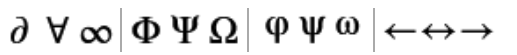• Select appropriate math formats from the Math Formats drop-down list.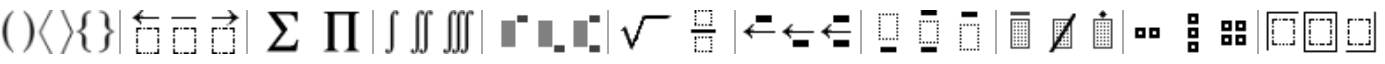3. Press the Right or Left Arrow key to move the insertion point. You can also click your desired insertion point.
4. Use the Zoom In or Zoom Out icons to enlarge or reduce the size of the equation. You can also scroll the mouse wheel while holding down the CTRL key.
5. When your equation is complete, press OK. The equation will be placed at the center of the current document.

#### To Insert Symbols Not Available in the Drop-Down List:

1. Click the Insert Symbol icon.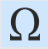2. In the Insert Symbol dialog box, select a category from the drop-down menu.
3. Do one of the following:
• Select a symbol and press Insert.
• Double-click on a symbol.
4. Repeat steps 2 and 3 as needed.
5. Click Close to close the dialog box.

#### To Insert Symbols by Specifying Their Unicode Values:

1. Click the Insert Symbol by Unicode Value icon.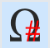2. Select a Unicode value type from the drop-down menu.
3. Type the Unicode value of the symbol you want to insert.
4. Click Insert.
5. Repeat steps 3 and 4 as needed.
6. Click Close to close the dialog box.

#### To Clear the List of Inserted Symbols:

Click the Trash icon located at the right side of the Insert Symbol by Unicode Value icon.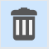#### To Adjust the Size of a Math Equation:

1. Select the equation object.
2. Do one of the following:
• Shift+drag one of the corners of the equation object to retain the width and height proportionally.
• Select Scale Proportional from the drop-down menu in the Properties bar and set the Width and Height.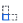You can restore the equation to its default size by clicking Restore Original Size in the Properties bar.

#### To Change the Color of a Math Equation:

1. Select the equation object.
2. Do one of the following:
• In the Properties bar, choose a color from the Equation Color drop-down menu.
• Apply another Fill color from the Toolbox.

#### To Edit a Math Equation:

1. Select the equation object.
2. In the Properties bar, click the Edit Equation button. You can also double-click an equation object to open the Math Equation Creator dialog box.
3. Edit the equation.
4. After finishing, click OK.
• # Related Articles

• ## Creating Math Equations

Creating Math Equations You can create editable math equation objects and place them in your documents. To Create a Math Equation: Choose Object | Create Math Equation. Create an equation in the Math Equation Creator dialog box by doing any of the ...
• ## Creating Custom Image Filters

Creating Custom Image Filters You can create your own special-effect and image-correcting filters using the Custom command. You can also save custom filters and use them in future Canvas X Draw documents. Filters work with an image one pixel at a ...
• ## Creating Custom Image Filters

Creating Custom Image Filters You can create your own special effect and image-correcting filters using the Custom command. You can also save custom filters and use them in future Canvas X Draw documents. Filters work with an image one pixel at a ...
• ## Creating Custom Image Filters

Creating Custom Image Filters You can create your own special effect and image-correcting filters using the Custom command. You can also save custom filters and use them in future Canvas X Draw documents. Filters work with an image one pixel at a ...
• ## Using Math Expression 2-D Plot Commands

Using Math Expression 2-D Plot Commands In Canvas X Draw you can use Math Expressions to create a visual representation of an equation. The visual representation is created as a vector object that uses the current Pen and Fill inks. You can also ...